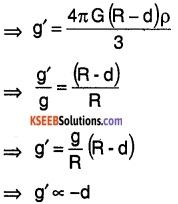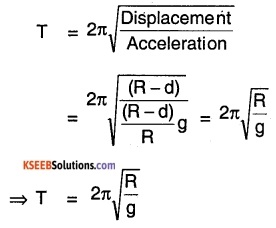# 1st PUC Physics Question Bank Chapter 14 Oscillations

## Karnataka 1st PUC Physics Question Bank Chapter 14 Oscillations

### 1st PUC Physics Oscillations Textbook Questions and Answers

Question 1.
Which of the following examples represent periodic motion?
(a) A swimmer completing one (return) trip from one bank of a river to the other and back.
(b) A freely suspended bar magnet displaced from its N-S direction and released.
(c) A hydrogen molecule rotating about its center of mass.
(d) An arrow released from a bow.
(b) and (c)

Question 2.
Which of the following examples represent (nearly) simple harmonic motion and which represent periodic but not simple harmonic motion?

1. the rotation of earth about its axis.
2. motion of an oscillating mercury column in a U-tube.
3. motion of a ball bearing inside a smooth curved bowl, when released from a point slightly above the lowermost point.
4. general vibrations of a poly-atomic molecule about Its equilibrium position.

1. Periodic but not SHM (Simple Harmonic Motion)
2. SHM
3. SHM
4. Periodic but not SHMQuestion 3.
The figure below depicts four x-t plots for the linear motion of a particle. Which of the piots represent periodic motion? What is the period of motion (in case of periodic motion)?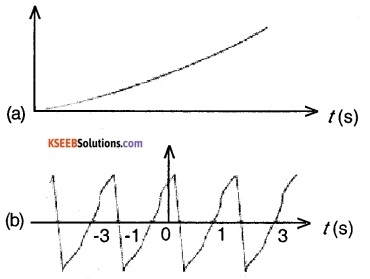(b) and (d) are in periodic motion with period of 2 sec.

Question 4.
Which of the following functions of time represent
(a) simple harmonic,
(b) periodic but not simple harmonic, and
(c) non-periodic motion?
Give period for each case of periodic motion (ω is any positive constant):
(a) sin ω t – cos ω t
(b) sin3 ω t
(c) 3 cos( π/4 – 2 ω t)
(d) cos ω t + cos 3 ω t + cos 5 ω t
(e) exp (- ω2 t2)
(f) 1 + ω t+ ω2 t2
(a) sin ω t – cos ω t = $$\sqrt{2}\left(\frac{1}{\sqrt{2}} \sin \omega t-\frac{1}{\sqrt{2}} \cos \omega t\right)$$
= $$\sqrt{2} \sin (\omega t-\pi / 4)$$ ; SHM , period = $$\frac{2 \pi}{\omega}$$
(b) sin3 ω t = $$\frac{1}{4}$$ (3 sin ωt – sin 3ωt) both terms are in SHM hence sin3 ωt is periodic.
period = $$\frac{2 \pi}{\omega}$$
(c) 3 cos (π/4 – 2 ω t) S H M with period = $$\frac{\pi}{\omega}$$
(d) cos ω t + cos 3 ω t + cos 5 ω t Superposition of 3 periodic motion,period = $$\frac{2 \pi}{\omega}$$
(e) exp (- ω2 t2) non periodic motion
(f) 1 + ω t+ ω2 t2 non periodic motion

Question 5.
A particle is in linear simple harmonic motion between two points, A and B, 10 cm apart. Take the direction from A to B as the positive direction and give the signs of velocity, acceleration, and force on the particle when it is
(a) at the end A,
(b) at the end B,
(c) at the mid-point of AB going towards A,
(d) at 2 cm away from B going towards A,
(e) at 3 cm away from A going towards B, and
(f) at 4 cm away from B going towards A.Question 6.
Which of the following relationships between the acceleration α and the displacement x of a particle involve simple harmonic motion?

1. α = 0.7x
2. α = -200x2
3. α = -10x
4. α = 100x3

α = -10 x (∵ acceleration is proportional and opposite to displacement)Question 7.
The motion of a particle executing simple harmonic motion is described by the displacement function, x(t) = A cos (ω t + Φ). If the initial (t = 0) position of the particle is 1 cm and Its initial velocity is ω cm/s, what are its amplitude and initial phase angle? The angular frequency of the particle is π s-1. If instead of the cosine function, we choose the sine function to describe the SHM: x = B sin (ω t + α), what are the amplitude and initial phase of the particle with the above initial conditions.
Given: x(t) = A cos(ω t + Φ) ……. (1)
at t = 0, x(0) = 1cm, ω = π s-1 ,
substituting in (1) we get
1 cm = A cos (0 × π + Φ)
⇒ A cos Φ =1     …… (2)
Differentiate equation (1) w.r.t. t
⇒ $$\frac{\mathrm{d} \mathrm{x}}{\mathrm{dt}}$$ – A ω sin (ω t + Φ)    …… (3)
at t = 0, v = ω cm /s, ω = π s-1
Substituting in 3
⇒ ω = – A ωsin Φ
⇒ 1 = – Asin Φ     ….. (4)
Divide equation (4) by (2)
⇒ 1 = $$\frac{-\sin \phi}{\cos \phi}$$
⇒ tan Φ = -1
⇒ Φ = $$\frac{-\pi}{4}$$
Φ = initial phase.
Substitute Φ value in equation (2) we get
1 = A cos $$\left(\frac{-\pi}{4}\right)$$
⇒ A = $$\sqrt{2} \mathrm{cm}$$
If x (t) = B sin (ω t + α)        ……. (5)
at t = 0,  x = 1 cm, ω = π s-1
Substituting in (5) we get
1 = B sin(α)                 …… (6)
Differentiate (5) w.r.t. ‘ t’
⇒ v = $$\frac{\mathrm{d} \mathrm{x}}{\mathrm{dt}}$$= B ω cos (ω t + α) dt
at t = 0, v = ω = π
⇒ 1 = B cos(α)           …… (7)
Divide(7) by (6)
we get tan Φ = 1
⇒ Φ = π/4 is the initial phase
Substitute Φ in equation (6) we get
1 = B sin (π/4) ⇒ B = $$\sqrt{2} \mathrm{cm}$$

Question 8.
A spring balance has a scale that reads from 0 to 50 kg. The length of the scale is 20cm. A body suspended from this balance, when displaced and released, oscillates with a period of 0.6 s. What is the weight of the body?
Maximum length Ymax = 0.2m
Maximum weight Mmax = 50 kg
We know that,
F = Mg = ky, at M = Mmax, Y = Ymax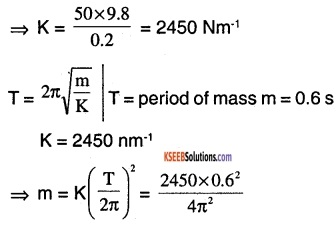⇒ m = 22.36 kg
Weight of the body = 22.36 × 9.8 = 219.1 N

Question 9.
A spring having a spring constant. 1200 N m-1 is mounted on a horizontal table as shown in Fig. A mass of 3 kg is attached to the free end of the spring. The mass Is then pulled sideways to a distance of 2.0 cm and released.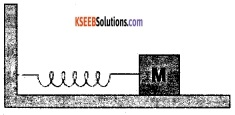Determine

1. the frequency of oscillations,
2. maximum acceleration of the mass, and
3. the maximum speed of the mass.

Given:
K = 1200 Nm-1, m = 3 kg, a = 2 cm
1.  Frequency of Oscillation
We know that T = $$2 \pi \sqrt{\frac{\mathrm{m}}{\mathrm{K}}}=2 \pi \sqrt{\frac{3}{1200}}$$.
⇒ T = 0.3144 s
⇒ f = $$\frac{1}{\mathrm{T}}$$ = 3.18/s

2. Maximum acceleration of mass (αmax) maximum acceleration is obtained when y = a
⇒ m αmax = Ka
⇒ αmax = $$\frac{\mathrm{Ka}}{\mathrm{m}}=\frac{1200 \times 0.02}{3}$$ = 8 ms-2

3. Maximum speed of the mass
Vmax = a ω = $$a \sqrt{\frac{k}{m}}=0.02 \times \sqrt{\frac{1200}{3}}$$
Vmax = 0.4 ms-1

Question 10.
In Exercise 14.9, let us take the position of mass when the spring is unstretched as x=0, and the direction from left to right as the positive direction of x-axis. Give x as a function of time t for the oscillating mass if at the moment we start the stopwatch (t= 0), the mass is
1. at the mean position,
2. at the maximum stretched position, and
3. at the maximum compressed position.
In what way do these functions for SHM differ from each other, in frequency, in amplitude or the Initial phase?
Given a = 2 cm k = 1200Nm-1 m = 3 kg
⇒ $$\omega=\sqrt{\frac{\mathrm{k}}{\mathrm{m}}}=\sqrt{\frac{1200}{3}}=20 \mathrm{s}^{-1}$$
let equation of S H M ⇒ x = A sin ω t
1. time is measured from mean position.
x = A sin ω t
⇒ x = 2 sin 20 t

2.  at maximum structured position phase angle is π/2
⇒ x = a sin (ω t + π/2)
⇒ x = 2 cos (20t)

3. At maximum compressed position phase angle is 3 π/2
x= a sin (ω t + 3 π/2)
⇒ x = – a cos ω tQuestion 11.
The figure below correspond to two circular motions. The radius of the circle, the period of revolution, the initial position, and the sense of revolution (i.e. clockwise or anticlockwise) are indicated on each figure.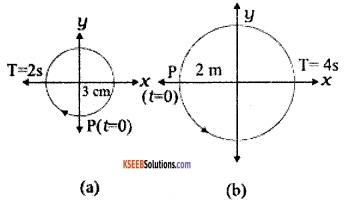Obtain the corresponding simple harmonic motions of the x-projection of the radius vector of the revolving particle P, in each case.(a) Let A be any point on the circle shown in fig (a). Draw AM ⊥ to x-axis. Point M refers to x – projection of the radius vector.
Now, ∠POA = θ = ωt, T = 2s OA = 3cm ⇒ ∠OAM =θ=ωt (∵ Alternate angles)⇒ x = 3 sin $$\frac{2 \pi}{\mathrm{T}}$$t(cm)
⇒ x = – 3 sin π t (cm)

(b) let A be any point on the circles of fig. (b). From A draw AM ⊥ to x-axis
Now ∠MOA= θ = ωt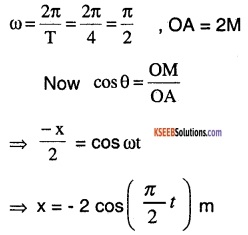Question 12.
Plot the corresponding reference circle for each of the following simple harmonic motions. Indicate the Initial (t =0) position of the particle, the radius of the circle, and the angular speed of the rotating particle. For simplicity, the sense of rotation may be fixed to be anti-clockwise In every case: (x Is In cm and t is in s).

1. x = – 2 sin (3t + π/3)
2. x = cos(π/6 – t)
3. x = 3 sin (2 π t + π/4)
4. x = 2 cos π t

1.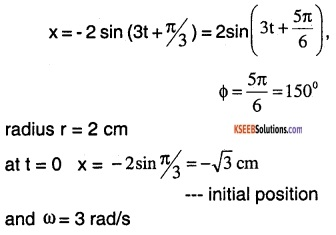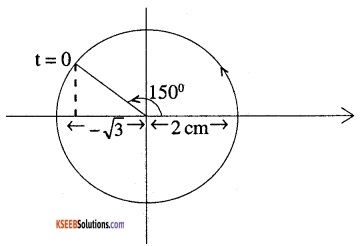2.  x = cos(π/6 – t)
at t = 0, x = cos (π/6) = $$\frac{\sqrt{3}}{2} \mathrm{cm}$$,
Φ = – π/6 = – 30°
ω = 1 rad / s3.4. x = 2 cos π t
r = 2 cm
ω = π rad / s
at t = 0 , x = 2 cm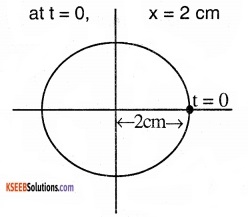Question 13.
Figure (a) shows a spring of force constant k clamped rigidly at one end and a mass m attached to its free end. A force F applied at the free end stretches the spring. Figure (b) shows the same spring with both ends free and attached to a mass m at either end. Each end of the spring in Fig. (b) is stretched by the same force F.1. What is the maximum extension of the spring in the two cases?
2. If the mass in Fig. (a) and the two masses in Fig. (b) are released, what is the period of oscillation in each case?

1. Consider figure (a)
let y be the extension produced in the spring F = ky
consider fig (b) each mass acts as if it is fixed w.r.t the other
⇒ F = ky  ⇒ y = F/k

2. consider fig (a)
F = – ky
ma = – ky   a = $$\frac{-\mathrm{k}}{\mathrm{m}} \mathrm{y}$$
⇒ ω2 = $$\frac{\mathrm{k}}{\mathrm{m}}$$
Therefore, $$\mathrm{T}=\frac{2 \pi}{\omega}=2 \pi \sqrt{\frac{\mathrm{m}}{\mathrm{k}}}$$
Consider fig (b)
let us assume (1) as centre of system and 2 springs each of length 1/2 attached to two masses. So k’ is the spring factor of each spring.
k’ = 2k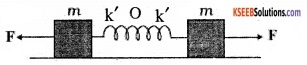⇒ $$\mathrm{T}=\frac{2 \pi}{\omega}$$
$$\mathrm{T}=2 \pi \sqrt{\frac{\mathrm{m}}{2 \mathrm{k}}}$$ is the period of oscillation in the case of (b).

Question 14.
The piston in the cylinder head of a locomotive has a stroke (twice the amplitude) of 1.0 m. If the piston moves with simple harmonic motion with an angular frequency of 200 rad/ min, what is its maximum speed?
Stroke = 1 m
Amplitude = $$\frac{\text { stroke }}{2}$$ = 1/2 m
ω = 200 rad / min
Vmax = ωA
= 200 × 1/2
= 100 m / min
Vmax = 1.67 m/s

Question 15.
The acceleration due to gravity on the surface of moon is 1.7 m s-2. What is the time period of a simple pendulum on the surface of moon if its time period on the surface of earth is 3.5 s? (g on the surface of earth is 9.8 m s-2)
gm =1.7 ms-2  ge = 9.8 ms-2  Te = 3.5 sQuestion 16.

1. Time period of a particle in SHM depends on the force constant k and mass m of the particle: T =$$2 \pi \sqrt{\frac{m}{k}}$$ . A simple pendulum executes SHM approximately. Why then is the time period of a pendulum independent of the mass of the pendulum?
2. The motion of a simple pendulum is approximately simple harmonic for small-angle oscillations. For larger angles of oscillation, a more involved analysis shows that T is greater than $$2 \pi \sqrt{\frac{1}{g}}$$ . Think of a qualitative argument to appreciate this result.
3. A man with a wristwatch on his hand falls from the top of a tower. Does the watch give correct time during the free fall?
4. What is the frequency of oscillation of a simple pendulum mounted in a cabin that is freely falling under gravity?

1. In case of a simple pendulum, k is directly proportional to m. Hence ratio of m / k is a constant. Hence time period doesn’t depend on mass.

2. Restoring force that brings body of pendulum back to its mean position F = – mg sin θ
Form small θ, sin θ ≈ θ = $$\frac{\mathrm{y}}{1}$$If approximation for sin θ = θ is not taken into account then Time period T > $$2 \pi \sqrt{\frac{1}{\mathrm{g}}}$$ This happens when θ is not small.

3. Wristwatch work on the principle of spring action. Hence acceleration due to gravity plays no role in the functioning of the wristwatch hence it gives correct time during free fall.

4. During free-fall acceleration, due to gravity is zero hence the pendulum will not vibrate (i.e.: frequency of oscillation is zero).Question 17.
A simple pendulum of length I and having a bob of mass M is suspended in a car. The car is moving on a circular track of radius R with a uniform speed v. If the pendulum makes small oscillations In a radial direction about its equilibrium position, what will be its time period?
Body of the pendulum is under the action of two accelerations, acceleration due to gravity ‘g’ and centripetal acceleration $$\alpha=\frac{v^{2}}{R}$$
effective acceleration = $$\alpha^{\prime}=\sqrt{\alpha^{2}+g^{2}}$$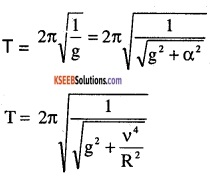Question 18.
A cylindrical piece of cork of density of base area A and height h floats in a liquid of density $$\rho_{\mathrm{t}}$$. The cork is depressed slightly and then released. Show that the cork oscillates up and down simple harmonically with a $$\mathrm{T}=2 \pi \sqrt{\frac{\mathrm{h} \rho}{\rho_{1} \mathrm{g}}}$$ where ρ is the density of cork. (Ignore damping due to viscosity of the liquid).
Initially in equilibrium,
weight of cork = weight of water displaced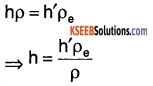When cork is pushed in, then the restoring force acting on it is :
f = – weight of the portion dipped after pushing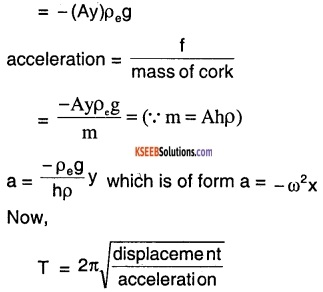Question 19.
One end of a U-tube containing mercury is connected to a suction pump and the other end to atmosphere. A small pressure difference is maintained between the two columns. Show that, when the suction pump is removed, the column of mercury in the U-tube executes simple harmonic, motion.
Restoring force acting on the liquid when suction pump is removed is,
f = – mg  ⇒ f= -(A × 2y)ρ × g
where, A = Cross – section area of U tube
ρ = density of liquid
⇒ f = – 2Aρgy
acceleration produced in liquid column
a = f/mass of liquid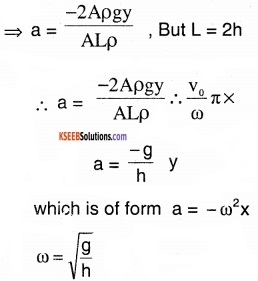hence it is a SHM.

Question 20.
An air chamber of volume V has a neck area of cross-section into which a ball of mass m just fits and can move up and down without any friction. Show that when the ball is pressed down a little and released, it executes SHM. Obtain an expression for the time period of oscillations assuming pressure-volume variations of air to be isothermal.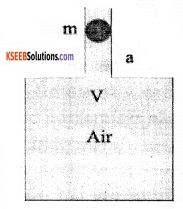Volume = V
mass of ball = m
cross-sectional Area = A
initial pressure on either side of the ball = atmospheric pressure = P
Let the charge in volume of air when ball is pressed be ∆V
∆V = Ay (y = displacement)
Now, Bulk modulus of elasticity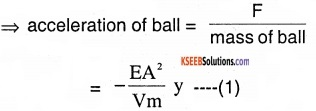The above equation is of the form,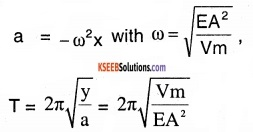hence it is in SHM.

Question 21.
You are riding in an automobile of mass 3000 kg. Assuming that you are examining the oscillation characteristics of Its suspension system. The suspension sags 15 cm when the entire automobile is placed on it. Also, the amplitude of oscillation decreases by 50% during one complete oscillation. Estimate the values of

1. the spring constant k and
2. the damping constant b for the spring and shock absorber system of one wheel, assuming that each wheel supports 750 kg.

1. Total mass = 3000 kg
mass supported by each wheel = 750 kg
y = 0.15 m
We know that,
mg = kg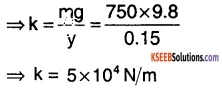2.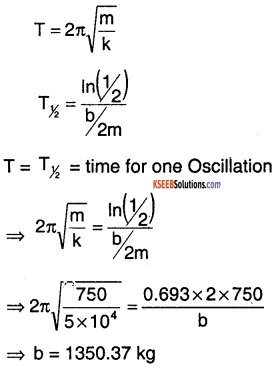Question 22.
Show that for a particle in linear SHM the average kinetic energy over a period of oscillation equals the average potential energy over the
same period.
Let the particle executing SHM starts from mean position.
Displacement can be given by
x = Asin ω t
Velocity v = A ω cosωt
Kinetic energy over one complete cycle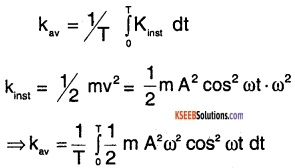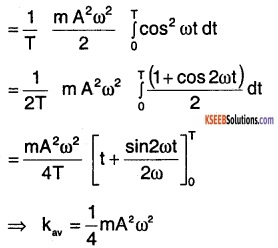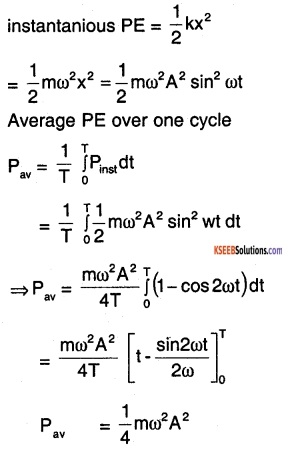Question 23.
A circular disc of mass 10 kg is suspended by a wire attached to its centre. The wire is twisted by rotating the disc and released. The period of torsional oscillators is found to be 1.5 s. The radius of the disc is 15 cm. Determine the torsional spring constant of the wire. (Torsional spring constant α is defined by the relation J= – α θ, where j is the restoring couple and θ the angle of twist).
We know that,Question 24.
A body describes simple harmonic motion with an amplitude of 5 cm and a period of 0.2 s. Find the acceleration and velocity of the body when the displacement is

1. 5 cm
2. 3 cm
3. 0 cm.

Given,
r = 5 cm
T = 0.2 s
$$\omega=\frac{2 \pi}{T}=\frac{2 \pi}{0.2}=10 \pi \mathrm{rad} / \mathrm{s}$$
acceleration A = – ω2y
velocity V = $$\omega \sqrt{r^{2}-y^{2}}$$
1. y = 5 cm
A = – (10π)2 × 0.05
⇒ A = 0.493 m / s2
V = $$10 \pi \sqrt{(0.05)^{2}-(0.05)^{2}}=0$$

2. y = 3 cm
A = (10π)2 × 0.03
⇒ A = – 0.296 m / s2
V= $$10 \pi \sqrt{(0.05)^{2}-(0.03)^{2}}$$
V = 0.4 πm/s

3. y = 0 cm
A = 0
V= $$10 \pi \sqrt{(0.05)^{2}-0}$$
= 0.5 πm/sQuestion 25.
A mass attached to a spring is freeto oscillate, with angular velocity ω, in a horizontal plane without friction or damping. It is pulled to a distance x0 and pushed towards the centre with a velocity v0 at time t = 0. Determine the amplitude of the resulting oscillations in terms of the parameters ω, x0 and v0.
[Hint : Start with the equation x = a cos (ωt+θ) and note that the initial velocity is negative.]
Let x = a cos (ωt + θ)
∴ v = $$\frac{d x}{d t}$$ = – a ω sin (ωt + θ)
When t = 0, x = x0 and $$\frac{d x}{d t}$$ = – v0
⇒ xo = a cos θ ….. (1) and
– v0 = – a ω sin θ
⇒ a sin θ = $$\frac{\mathrm{v}_{0}}{\omega}$$ …..(2)
Squarring and adding (1) and (2),
a2 = $$=x_{0}^{2}+\frac{v_{0}^{2}}{\omega^{2}} \Rightarrow a=\sqrt{x_{0}^{2}+\frac{v_{0}^{2}}{\omega^{2}}}$$

### 1st PUC Physics Oscillations One Mark Questions and Answers

Question 1.
Mention the relation between period and frequency of periodic motion.
Frequency f = $$\frac{1}{T}$$

Question 2.
A particle executes simple harmonic motion. At what point on its path is the acceleration maximum?
Acceleration is maximum at a point of maximum displacement from the mean position.

Question 3.
At what position the KE of an oscillating simple pendulum Is maximum?
Kinetic energy is maximum at the mean position.

Question 4.
What is the condition for motion of a particle to be SHM?
Acceleration should be proportional to displacement and always directed towards mean position.

Question 5.
What is Oscillation?
To and for motion in the same path is called Oscillation.

Question 6.
How will the period of a simple pendulum change when its length is doubled?
Tnew = $$\sqrt{2}$$ ToldQuestion 7.
Will a pendulum’s time period increases or decreases when taken to the top of the mountain?
Increases, g decreases as high altitude and $$\mathrm{T} \alpha \frac{1}{\sqrt{\mathrm{g}}}$$

Question 8.
Two simple pendulum of equal length cross each other at mean position. What is their phase difference?

Question 9.
How many times in one vibration, KE and PE become maximum?
Two.

Question 10.
When is the tension maximum in the spring of a simple pendulum?
Mean position.

Question 11.
A spring of spring constant k Is cut Into two equal parts. What is the spring constant of each part?
2 K

Question 12.
What Is the phase difference between the displacement and velocity in a SHM?

Question 13.
State force law for a SHM.
Force, F = mω2x
m = mass
ω = angular speed
x = displacement

Question 14.
A pendulum is making one oscilla¬tion in every two seconds. What is the frequency of oscillation?
f = $$\frac{1}{T}=\frac{1}{2} s^{-1}$$Question 15.
What is the frequency of oscillation of a simple pendulum mounted in a cabin that is freely falling under gravity?
Frequency is 0 because the acceleration zero during free fall.

Question 16.
A simple pendulum Is inside a spacecraft. What should be its time period vibration?
Pendulum does not oscillate.

Question 17.
What are isochronous vibrations?
When the time period is independent of amplitude such an oscillation is called isochronous.

### 1st PUC Physics Oscillations Two Marks Questions and Answers

Question 1.
Define simple harmonic motion (SHM). Give an example.
A particle is said to have SHM if the acceleration of the particle is directly proportional to its displacement from the mean position and directed towards the mean position.
E.g.:

1. Vertical oscillations of a loaded spring.
2. Oscillations of bob of a simple pendulum.
3. Vibrations of string of musical instruments.
4. Motion of air particles during the propagation of sound waves.
5. Vibration of a tuning fork.

Question 2.
Mention expression for velocity and acceleration of a particle executing SHM.
Velocity of a particle executing SHM is v = $$\omega \sqrt{A^{2}-y^{2}}$$
Acceleration of a particle executing SHM is a = – ω2y
y is the displacement of a particle from its mean position in t seconds. A is the amplitude and ω is the angular frequency.

Question 3.
Mention expression for K.E, P.E and total energy of a particle executing SHM.
Potential energy of particle at any instant t second is Ep = 1/2 m ω2 y2.
Kinetic energy of particle at any instant t second is, EK = 1/2 m ω2 (A2 – y2).
Total energy of particle at any instant is constant E = EK + Ep = 1/2 m ω2 A2.
A is the amplitude and w is the angular frequency and m is mass of the particle y is the displacement of a particle from its mean position in t seconds.Question 4.
The acceleration of a particle executing SHM Is 20ms-1 at a distance of 5 m from the mean position. Calculate its time period and frequency.
a = 20m/s2, y = 5m.
We know that a = | ω2 y |
⇒ $$\omega=\sqrt{\frac{a}{y}}=\sqrt{\frac{20}{5}}$$= 2 rad/s or 2πf = 2
Frequency f = $$\frac{2}{2 \times 3.14}$$ = 0.3185 Hz
Period T = $$\frac{1}{f}=\frac{1}{0.3185}$$ = 3.14s

Question 5.
Differentiate between forced oscillations and resonance.
1. Forced Oscillations:
A body oscillates with the help of external periodic force with a frequency different from natural frequency of body.

2. Resonance:
A body oscillating with its natural frequency with the help of external periodic force whose frequency is equal to natural frequency of body.

Question 6.
Two linear, simple harmonic motion of equal amplitudes and frequencies ω and 2ω are impressed on a particle along the axis of X and Y respectively. If the initial phase difference is π/2, then find the resultant path followed by particle
Let
X = Asinωt …… (1)
Y= Acos2ωt …….(2)
(1) and (2) represent SHM with equal amplitude and phase difference π/2 with frequencies ω and 2ω.

Question 7.
Derive an expression for K.E and P.E of a particle SHM.
We know that, Potential energy
U = 1/2 kx2
For a particle in SHM, k = mω2 and x = Asin ωt
⇒ U = 1/2 mω2 A2 sin2 ωt
Kinetic energy, k= 1/2 mv2
now, v = ωy = ωA cos ωt
⇒ K= 1/2 mω2 A2cos2ωt

Question 8.
The amplitude of a oscillating simple pendulum Is doubled. What will be its effect on

1. periodic time
2. total energy
3. maximum velocity

1. Periodic time does not change. T is independent of amplitude.
2. Total energy, T.E = 1/2 mω2 A2
A is doubled ⇒ T.Enew = 4 TEold
3. Maximum velocity, Vmax = ωA
A is doubled ⇒ Vmax is doubled.

Question 9.
The frequency of oscillations of a mass m suspended by a spring is V1. If the length of spring is cut to one half, the same mass oscillates with frequency V2. Calculate V2/V1.
V1 = $$\frac{1}{2 \pi} \sqrt{\frac{k}{m}}$$
where K is the spring constant and m is the mass
V2 = $$\frac{1}{2 \pi} \sqrt{\frac{2 \mathrm{k}}{\mathrm{m}}}$$
∴ $$\frac{V_{2}}{V_{1}}=\sqrt{2}$$Question 10.
Discuss some important characteristics of wave motion.

1. Wave transports energy.
2. particles are not transported.
3. Elasticity and inertia determine the motion of particle distribution.

Question 11.
Differentiate between free oscillations and forced oscillations with the help of examples.
Consider a pendulum in free space (without air) it oscillates freely. This is free oscillation. Consider another pendulum in a viscous liquid it oscillates only if there is external force this is forced oscillation.

Question 12.
List any two characteristics of SHM.

1. SHM is always directed towards mean position.
2. Acceleration is directly proportioned to displacement but opposite in direction.

### 1st PUC Physics Oscillations Three Marks Questions and Answers

Question 1.
Define the terms

1. amplitude
2. period
3. frequency
4. phase related with a particle executes SHM.

1. Amplitude:
Maximum displacement of the particle from the mean position is called amplitude.

2. Period :
Time taken by the particle to complete one oscillation is called time period. (T)

3. Frequency :
The number of oscillations completed by the particle in one second is called frequency (f)

4. Phase:
Phase of a particle is defined as the fraction of the time period that has elapsed since the particle last passed through its mean position in the positive direction.Question 2.
For an oscillating pendulum, establish the relation $$\frac{d^{2} \theta}{d t^{2}}=-\omega^{2} \theta$$ where $$\omega=\sqrt{\frac{g}{1}} \theta$$ = small angular displacement.restoring force Fr = – mg sin θ
torque on pendulum $$\tau=\mathrm{I} \alpha=\mathrm{ml}^{2} \alpha$$
restoring torque = torque on pendulumWe know that = $$\frac{\mathrm{d}^{2} \theta}{\mathrm{dt}^{2}}=\alpha$$ and $$\omega=\sqrt{\frac{g}{1}}$$
⇒ $$\frac{\mathrm{d}^{2} \theta}{\mathrm{dt}^{2}}=-\omega^{2} \theta$$

Question 3.
A body of mass 1 kg is suspended from a weightless spring having force constant 600 Nm-1. Another body of mass 0.5 kg moves vertically upwards lift the suspended body with a velocity of 3 ms-1 and tets embedded in it. Find the frequency of oscillation and amplitude of motion.
Total mass = (1 + 0.5)kg = 1.
k = 600 nm-1
frequency of oscillations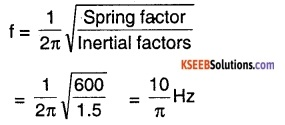Let V1 be velocity of mass after collision
⇒ m1 V2 = (m1 + m2,) V1
⇒ 0.5 × 3 = 1.5 V1
⇒ V1 = 1 m/s
According to law of conservation of energy
(PE)max = (KE)max
$$\frac{1}{2} m v_{1}^{2}=1/2^{K A^{2}}$$
$$\frac{1}{2} 1.5 \times 1^{2}=\frac{1}{2} 600 \mathrm{A}^{2}$$
⇒ A = 5 cm

Question 4.
The bottom of a dip on a road has a radius of curvature R. A rickshaw of mass M left a little away from the bottom oscillates about this dip. Deduce an expression for the period of oscillation.Let the rikshaw of mass M be at any point P in the dip of radius R. Let O be the centre of this circular path. This case is similar to that of a simple pendulum and assume that e is small.
restoring force
F = -mg sinθ
F = – mg θ
displacement of rickshaw = R θQuestion 5.
Find an expression for body vibrating in SHM.
Let the SHM equation be
x = Asin ω tPotential energy is given by
= 1/2 kx2 = 1/2 m ω 2 k2
= 1/2 mω2 A2 sin2 ω t
Kinetic energy = 1/2 mv2
= 1/2 m ω 2 A2 cos2 ω t
Total energy    = PE + KE
= 1/2 mω2 A2 sin2 ω t + 1/2 mω2 A2 cos2 ω t
= 1/2 mω 2 A2

Question 6.
Show that when a particle is moving in SHM its velocity at a distance $$\frac{\sqrt{3}}{2}$$ its amplitude from the central position is half its velocity in central position.
For a particle in SHM
$$\mathrm{V}=\omega \sqrt{\mathrm{A}^{2}-\mathrm{x}^{2}}$$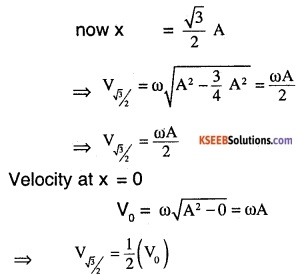Question 7.
A body oscillates with SHM according to the equation.
x(t) = 5cos (2π t + π /4) Where x is in meters and t is in seconds calculate,

1. displacement att = 0
2. Angular frequency
3. Vmax

1. displacement at t = 0
x(0) = 5cos(2π 0 + π/4)
⇒ x = $$\frac{5}{\sqrt{2}} m$$

2. Angular frequency

3. Vmax = aω = 2π × 5 = 10π m/sQuestion 8.
What is spring factor? Find its value in case of two springs connected in

1. series
2. Parallel

Spring factor (k):
Force acting for unit extension produced is called spring factor.
1. When two springs connected in series,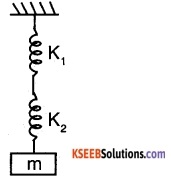force experienced by both springs is same.
Total extension (x) is sum of individual extension.
⇒ x = x1 + x2
$$\frac{F}{-K_{e q}}=\frac{F}{-K_{1}}+\frac{F}{-K_{2}}$$ ⇒ 1/Keq = 1/K1 + 1/K2
Keq = $$\frac{\mathrm{K}_{1} \mathrm{K}_{2}}{\mathrm{K}_{1}+\mathrm{K}_{2}}$$

2. When two springs are in parallel,Net force experienced is the sum of force experienced in both springs. But the extension will be the same.
Feq = F1 + F2
Keqx =K1 X + K2X
⇒ Keq = K1 + K2

Question 9.
Explain the relation in phase between displacement, velocity, and acceleration in SHM, graphically as well as theoretically.
Let displacement x = Asinωt
Velocity v= ω Acosω t
acceleration a = – ω2 Asin ω t = ω2 Asin(ω t + π )
Displacement and velocity have phase difference of π/2 radians.
v and α has $$\frac{\pi}{2}$$ radians of phase difference. α ans x has π radians of phase difference.
Assume ω >1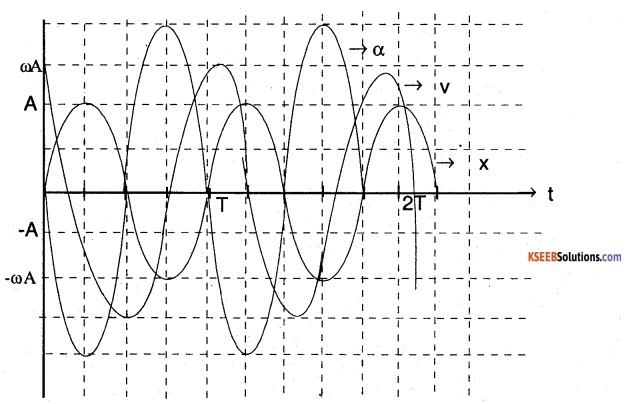Question 10.
For a particle in SHM, the displacement x of the particle at a function of time t is given as x = Asin(2πt) …..(1) where x is in centimeters and t is in seconds. Let the time taken by the particle to travel from x=0 to x = A/2 beT1 and the time taken to travel from x = A/2 to x = A be T2. Find T1/T2
ω =2π = $$\frac{2 \pi}{\mathrm{T}}$$ ⇒ T= 1 s
at t = 0 , x = 0
now if x = A/2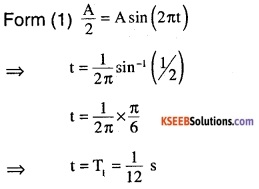now in a SHM time taken from 0 to A is $$\frac{T}{4}$$
⇒ time taken for x = A/2 to A is $$\frac{T}{4}$$ -T/12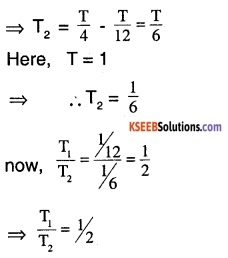Question 11.
What is SHM? Show that in SHM acceleration is directly proportional to Its displacement at a given instant.
Simple Harmonic Motion is a type of motion in which displacement is always directed towards mean position and acceleration is directly proportional to displacement and opposite in direction.
Let a SHM be represented by
x = Asin ωt
⇒ $$\frac{\mathrm{d} x}{\mathrm{dt}}$$ = A ω cos ωt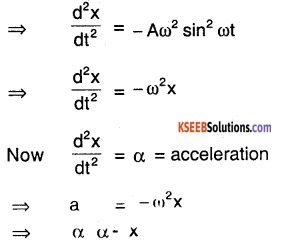acceleration is proportional to displacement and opposite in direction.

Question 12.
A 0.2 kg of mass hangs at the end of a spring. When 0.02 kg more mass is added to the end of the spring, it stretched 7cm more. If the 0.02 kg mass is removed, what will be the period of vibration of the system?
Length stretched x= 7 cm = 0.07 mTime period T = $$2 \pi \sqrt{\frac{\mathrm{m}}{\mathrm{k}}}$$Question 13.
A particle executes SHM of time period 10 sec. The displacement of particle at any instant is x = 10 sinωt(cm) Find

1. Velocity of body 2 s after it passes through mean position.
2. Acceleration of body 2 s after it passes mean position.

Given x = 10 sin ωt T = 10 sec
A = 10 cm   ω = $$\frac{2 \pi}{10}$$ rad/s
Let at t = 0 body be at the mean position.
Now at t = 2
1.  V = Aω cos ωt
= 10ω cos(ω2)cm/s
⇒ $$V=10 \times \frac{2 \pi}{10} \cos \left(\frac{2 \pi}{10} 2\right)$$
⇒ V = 1.94 cm/s

2. Acceleration
a = – Aω2 sinωt
$$= – 10 \times \frac{4 \pi^{2}}{100} \sin \left(\frac{4 \pi}{10}\right)$$
⇒ a = – 3.75 cm/s2Question 14.
What is a simple pendulum? Show that the motion of the pendulum is SHM and hence deduce an expression for the time period of pendulum. Also, define Second’s pendulum.
Simple pendulum is a point mass body suspended by a weightless thread or string from a rigid support about which it is free to oscillate.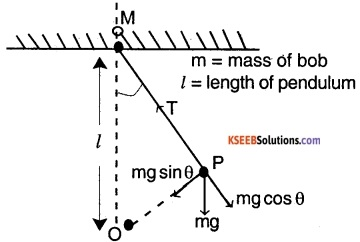Now restoring force acting on body can be given by
F = – mgsinθ (from figure)
If  θ is small
F= – mgθ    (∵ sin θ ≈ θ)
Let P be any point on the path of pendulum and makes ∠OMP = θ which is small and arc OP be x (displacement) then
$$\dot{\theta}=\frac{\mathrm{OP}}{1}=\frac{\mathrm{x}}{1}$$
⇒ F = $$\frac{-m g x}{1}$$ ……(1)
⇒ force ∝ displacement and opposite in direction hence an SHM
Now this of form F = -kx ……(2)
⇒ k = mg/l (from (1) and (2)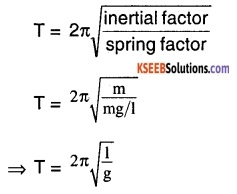Question 15.
A body of mass ‘m’ suspended from a spring executes SHM. Calculate the ratio of kinetic energy and potential energy of the body when it is at half the amplitude far from the mean position.
KE of a mass suspend from a spring
KE = mω2(a2 – y2)
Potential energy of a mass suspend from a spring
PE = 1/2 mω2 y2
$$\frac{\mathrm{KE}}{\mathrm{PE}}=\frac{\left(\mathrm{a}^{2}-\mathrm{y}^{2}\right) 2}{\mathrm{y}^{2}}$$
at y = a/2
⇒ $$\frac{\mathrm{KE}}{\mathrm{PE}}=\frac{\left(\mathrm{a}^{2}-\mathrm{a}^{2} / 4\right)^{2}}{\mathrm{a}^{2} / 4}$$
⇒ $$\frac{\mathrm{KE}}{\mathrm{PE}}=3$$

Question 16.
If x = a cos ωt + b sin ωt, show that it represents SHM.
We have,
x = a cos ωt + b sin ωt
Now, $$\frac{\mathrm{d} \mathrm{x}}{\mathrm{dt}}$$ = – aω sinωt + bω cosωt
$$\frac{\mathrm{d}^{2} \mathrm{x}}{\mathrm{dt}^{2}}$$ = – aω2 cos ωt + bω2 sin ωt
⇒ $$\frac{\mathrm{d}^{2} \mathrm{x}}{\mathrm{dt}^{2}}$$ = – ω2 (a cos ωt+ b sin ωt)
⇒ $$\frac{\mathrm{d}^{2} \mathrm{x}}{\mathrm{dt}^{2}}$$ = – ω2x
⇒ α = – ω2x
Hence an SHM

Question 17.
Find the expression for the total energy of a particle executing SHM.
For a SHM PE = 1/2 kx2
= 1/2 mω2 x2 = 1/2 mω A2 sin2 ωt
KE= 1/2 mv2
v = $$\frac{\mathrm{d} \mathrm{x}}{\mathrm{dt}}$$ = – Aω cos ωt
⇒ KE = 1/2 mA2ω2 cos2 ωt
Total energy = KE + PE = 1/2 mω2 A2 cos2 ωt + 1/2 mω2 sin2 ωt
Total energy = 1/2 mω2 A2

### 1st PUC Physics Oscillations Five Marks Questions and Answers

Question 1.

1. Find the total energy of particle executing SHM?
2. Show graphically the variation of PE and KE with time In SHM.
3. What is the frequency of these energies w.r.t the frequency of the particle executing SHM?

1. PE at any instant
PE = 1/2 kx2
= 1/2 mω2 A2 sin2 ωt
(∵ k = mω2 and x = A sin ωt)
⇒ PE = 1/2 mω2 A2 sin2 ωt
KE at any instant KE = 1/2 mv2
KE = 1/2 mA2 ω2 cos2 ωt
Total energy = KE + PE
⇒ Total energy = 1/2 mω2 A2sin2 ωt + 1/2 mω2 A2 cos2 ωt
⇒ Total energy = 1/2 mω2 A2

2. Graph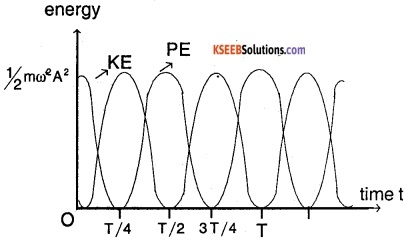3. Frequency = $$\frac{2}{\text { period }}=\frac{2}{\mathrm{T}}$$

1st PUC Physics Oscillations Numerical Problems Questions and Answers

Question 1.
A spring compressed by 0.1m develops a restoring force of 10N. A body of mass 4 kg is placed on it. Deduce the

1. force constant of the spring
2. depression of the spring under the weight of the body
3. period of oscillation, if the body is disturbed.

Given
Restoring force, F = 10N
Mass of the body, m = 4kg
displacement, x =0.1m

1. $$\mathrm{k}=\frac{\text { force }}{\text { displacement }}=\frac{10}{0.1}$$ = 100 Nm-1
2. Depression due to weight $$=\frac{\text { force }}{\mathbf{k}}=\frac{4 \times 10}{100}$$ = 0.4 m
3. Period of oscillation T = $$2 \pi \sqrt{\frac{m}{k}}$$ $$=2 \pi \sqrt{\frac{4}{100}}$$ = 0.4 πs
T = 0.4 πs

Question 2.
A vertical U- tube of uniform crosssection contains water up to a height to 20cm. Calculate the time period of the oscillation of water when it is disturbed.
The length of liquid column
L = 2 × 20cm = 40 cm
Time period of oscillation
$$=2 \pi \sqrt{\frac{L}{2 g}}=2 \pi \sqrt{\frac{40}{2 \times 9.80}}$$ = 0.9 sQuestion 3.
A cylindrical piece of cork of base area ‘A’ and height ‘h’ floats in a liquid of density $$\rho_{\mathrm{e}}$$. The cork is depressed slightly and then released. Show that the cork oscillates up and down simple harmonically with a period of $$\mathrm{T}=2 \pi \sqrt{\frac{\mathrm{h} \rho}{\rho_{\mathrm{e}} \mathrm{g}}}$$ where ρ is the density of cork. (Ignore damping due to viscosity of the liquid).
Let x be the depression created.
Excess upthrust caused is, Ue= g$$\rho_{\mathrm{e}}$$ Ax
Restoring force = U = gA$$\rho_{\mathrm{e}}$$ x
mass = m = Ahρ
Now F = mα;  α = acceleration
Ahρα = -gA$$\rho_{\mathrm{e}}$$ x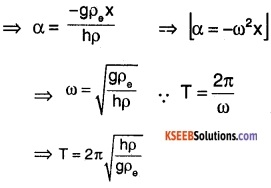Question 4.
If this earth were a homogenous sphere and a straight hole bored in it through its centre. Show that if a body were dropped into the hole it would execute a SHM. Also, find its time period.
Let mass of body dropped = m
mass of earth = M
mg = $$=\frac{\mathrm{GMm}}{\mathrm{R}^{2}}$$ ⇒  g = $$\frac{\mathrm{GM}}{\mathrm{R}^{2}}$$
⇒  $$\mathrm{g}=\frac{\mathrm{G}}{\mathrm{R}^{2}} \frac{4}{3} \pi \mathrm{R}^{2} \rho=\frac{4 \pi \mathrm{GR} \rho}{3}$$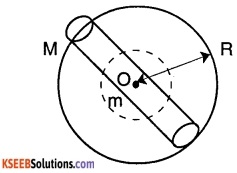$$\mathrm{g}^{\prime}=\frac{\mathrm{Gm}^{\prime}}{(\mathrm{R}-\mathrm{d})^{2}}$$